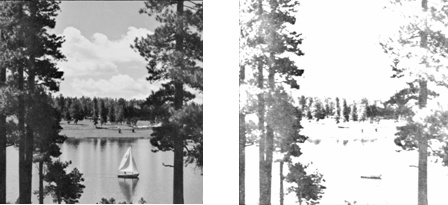There is no denying that the convolution operation has a rather persistent way of creeping into practically all of the statistical, electrical and computer engineering applications, pretty much in anything that involves signals and in finding a way of dealing with them. We’ve all got a bunch of formulas tucked away somewhere in our dusty old class notes, but this time, let’s try and take a closer look at what’s going on.

What we have already been taught is probably a mathematical statement involving functions and their integration. For those who need to know or recall what that looks like, here you go:

The convolution of the two functions, f and g, is fg. It is defined as the integral of the product of the two functions, provided one of them, could be f or g, is reversed and shifted. So basically, it’s like performing a kind of integral transform, on the functions, so the result gives us something different from either of the two.

Here’s an explanation of what that could actually mean. The convolution tells us the amount of overlap of one function (say f) as it is shifted over another function (say g).  That’s like taking the two of them, f and g, and sort of blending them. What that does is, it produces a third function (neither f nor g), that is modified version of one of the functions. This offspring function (say) gives us an idea of the overlap between the two parent functions, (f and g), as a function of the amount that one of the parent functions has shifted. So if we have the shift, or translation, in one coordinate axis, we visualize the overlap area on the other axis.

Need a clearer picture? This is to help with the visualization (Fig 2 & Fig. 3):Fig 2. Convolution of the boxcar signal with itselfFig. 3 Convolution of the spiky function with box signalFig. 4. Comparison of Convolution, Cross correlation and Auto correlationBy Cmglee (Own work) [CC-BY-SA-3.0 (http://creativecommons.org/licenses/by-sa/3.0) or GFDL (http://www.gnu.org/copyleft/fdl.html)], via Wikimedia Commons

Figure 4 shows the distinction between convolution and correlation functions.

The convolution can be defined for functions on groups other than the Euclidean coordinate space. We saw what the operation looked like for a continuous time domain. But we could have it defined for functions on a set of integers. That is termed as the discrete convolution, because we are dealing with discrete numbers there, not a continuous function variable. We could also have it defined for periodic functions, such as the discrete-time Fourier transform (DTFT), for which the convolution can be defined on a circle. We then call it a periodic convolution.

But again, we are left with the question, what is this about, really? Well simply because sometimes we need to modify our signals to look like, or sound like, or behave in a way that could enhance it to be better suitable for our work. The way we do this is multiply our waveform in the time domain with another, such that their frequency content, or spectra, gets multiplied in frequency domain. That means, any frequency which has a strong influence in both our signals will appear strong in convolved signal too, and the weak ones remain weak in the output.

Consider that we have a space with an impulse response. We could obtain the response by recording a short burst of sound in that space, something like a clap, or a balloon bursting in that space, giving us the idea of the reverberant characteristics of the space. Next, when we convolve any signal, with that particular impulse response, we will have a signal that seems to have been recorded in that space! If we convolve any sound with another sound, and not an impulse response, we “filter” one sound, through the spectrum of the other. As a result, the frequencies which the two have in common are accentuated. If we convolve the sound with itself, the frequencies which are already strong get highlighted and the ones which are weak, diminish in strength.

The convolution has a strong influence in image processing applications as well. In this case, we consider convolution as a neighborhood operation in which each output pixel is the weighted sum of neighboring input pixels. The matrix of weights, called the convolution kernel, is termed as filter.

If our image is this:

A =[17    24     1     8     15

23     5     7     14     16

4     6    13     20     22

10    12    19    21      3

11    18    25     2     9]

h = [8   1   6] is the kernel,

then

A*h= [-24    16    16   -14    8

-5    16    -9    -9    14

-6    -9   -14    -9    20

-12    -9    -9    16    21

-18   -14    16    16   2].

Changing these values of the pixels could have considerable impact on our image. Consider the result of convolving a 256*256 grayscale image with the kernel h = [ 1 1 1] (Fig 5):Fig. 5. a. Original image                                   b. Filtered image

As another example, the convolution of a sharp image with a lens function may produce a blurred or distorted image.

In electrical engineering, the convolution of the input signal with an impulse response gives the output of a linear time-invariant (LTI) system. So at a particular moment, the output is the resultant effect of all previous values of the input function. The impulse response provides the factor as a function of time elapsed since each input value came in.

In probability theory, the probability distribution of the sum of two independent random variables is the convolution of their individual distribution.

These are just a few of the examples. So here’s the cue to go discover how the convolution plays a significant role in our daily dealings with all the images we may see, sounds we may hear and data we may analyze.

Share.